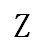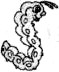• 试题检索 :
• 教材版本 :
• 课本 :
• 题型 :
• 难易度 :

zh　　ch 　sh　 c 　s

ie wu ai ye yu qiu xiao yue er ei hua

[ ]
A、KLMNOR
B、FHPMST
C、Q R S W Z
D、BDEK R

A—（）　d—（）　j—（）　q—（）　G—（）　R—（）
y—（）　T—（）　h—（）　M—（）　N—（）　b—（）

A（　　）　G（　　）　I（　　） 　H（　　）　T（　　）　Q（　　）
（　　）b　（　　）l　（　　）m 　（　　）r　（　　）n　（　　）d

1．______________________
2．______________________
3．______________________

A（ ）（ ） D（ ）（ ）G （ ）I（ ）（ ） L（ ）（ ）
O（ ）（） R（ ）（ ）（ ） V（ ）（ ） YZ

B　　P　　T　　F　　H　　E　　D　　A　　L　　S　　Y　　J
_________________________________________________________________________
_________________________________________________________________________________
________
________